NEW
Sequencing Props
The converse of III.27--about equal angles and arcs. And sequencing.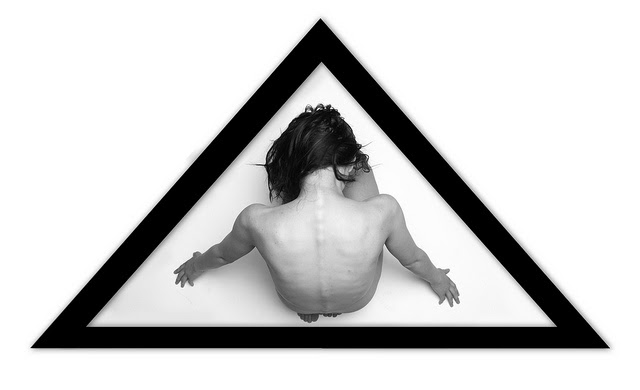Trapped in a Triangle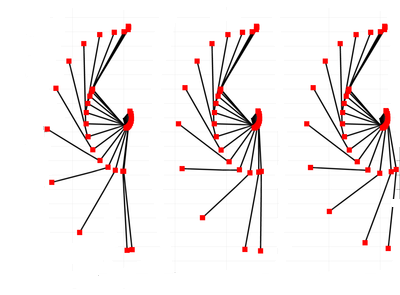Turning the Dials
Boring proof of III.27. Also, I F*cking Love Math.Perps and Diagonals
The sum of perpendiculars to diagonals in a rectangle.Stirring Up a Proof
A proof requiring just memory and practice.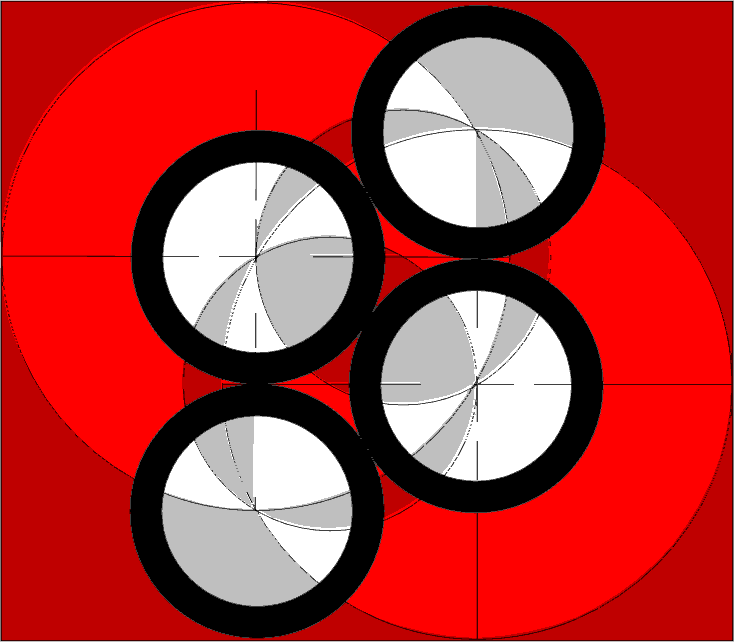Touching Circles
A seemingly obvious Proposition III.12 from the Elements.Proposition II.4
Animated setup for II.4. Then it peters out.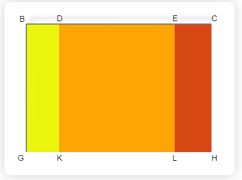The Distributive Property
Some interactive work with II.1, a geometric Distributive Property.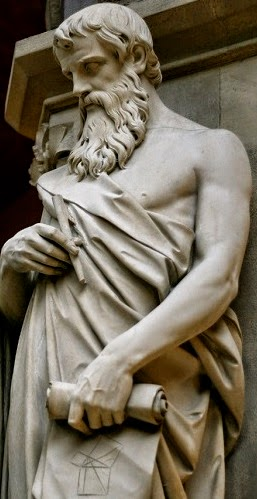Extending Pythagoras
Pappus' extension of I.47, the Pythagorean Theorem. And blobs.Combining Theorems
Three proofs in one (I.34, I.37, and I.41). And a simulation.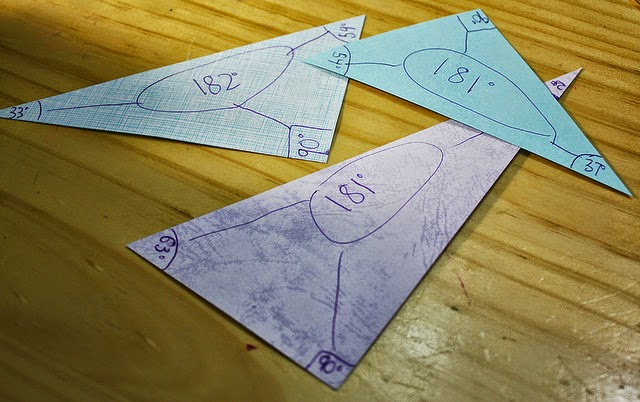Angles in a Triangle
Euclid's proof (I.32) that a triangle's angle measures have a sum of 180$$^\circ$$.Elegance Schmelegance
Proof of a 2005 Math Olympiad problem.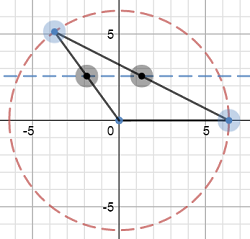$$\small\Delta$$ Midsegment Theorem
Proof using SAS and congruent corresponding angles.Varignon's Theorem
Interactive demo and proof using Midsegment Theorem.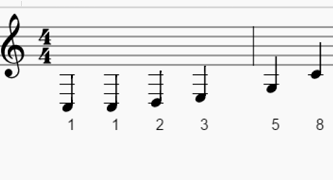Fibonacci Sequence
A musical pattern in the Fibonacci numbers.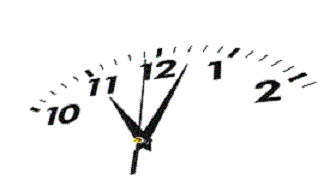Spacetime
Spacetime graphs and some basic ideas in special relativity.Alternate Interior $$\small\measuredangle$$s
Euclid's proof of I.27 and some discussion about 'transitional' topics in math.Secant Theorem
Proof using inscribed angles and AA similarity.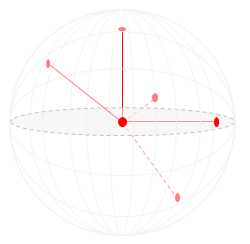Formula for Intensity
The formula is related to simple division and graphed.Side-Angle-Side
Euclid's proof of I.4 and some discussion about precision.Inscribed $$\small\measuredangle$$ Theorem
Proof involves 3 cases. Uses the base angle and exterior angle theorems.Pick's Theorem
Interactive demo and proof. Some notes about speculation in math.Vertical $$\small\measuredangle$$ Theorem
Proof of I.15 using I.13. Explains where vertical angles got their name.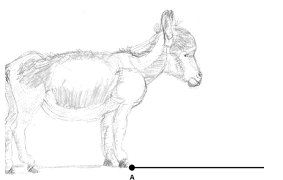$$\small\Delta$$ Inequality Theorem
Proof and discussion about intuition in mathematics.The Cissoid
Demo and explanation of the cissoid curve. Other stuff too.Angle-Side-Angle
Proof by contradiction of I.26. Some notes about how it was first used.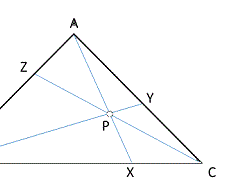Ceva's Theorem
Interactive proportionality demo. Then the proof.